# Mean State

Period Mean (original grids) [W/m2]
Model Period Mean (intersection) [W/m2]
Model Period Mean (complement) [W/m2]
Benchmark Period Mean (intersection) [W/m2]
Benchmark Period Mean (complement) [W/m2]
Bias [W/m2]
RMSE [W/m2]
Phase Shift [months]
Bias Score 
RMSE Score 
Seasonal Cycle Score 
Spatial Distribution Score 
Interannual Variability Score 
Overall Score 
Benchmark [-] 131.
CRUNCEPv7 [-] 138. 139. 130. 157. 9.19 21.4 1.29 0.461 0.457 0.834 0.990 0.511 0.618
GSWP3v1 [-] 118. 119. 130. 157. -11.1 20.8 1.31 0.564 0.427 0.824 0.990 0.678 0.652
WFDEI [-] 124. 124. 130. 157. -4.96 21.8 1.65 0.610 0.358 0.774 0.986 0.715 0.633
Period Mean (original grids) [W/m2]
Model Period Mean (intersection) [W/m2]
Model Period Mean (complement) [W/m2]
Benchmark Period Mean (intersection) [W/m2]
Benchmark Period Mean (complement) [W/m2]
Bias [W/m2]
RMSE [W/m2]
Phase Shift [months]
Bias Score 
RMSE Score 
Seasonal Cycle Score 
Spatial Distribution Score 
Interannual Variability Score 
Overall Score 
Benchmark [-] 123.
CRUNCEPv7 [-] 114. 114. 122. 160. -7.78 23.8 1.58 0.590 0.466 0.751 0.973 0.585 0.639
GSWP3v1 [-] 103. 102. 122. 160. -19.8 25.9 1.34 0.456 0.513 0.799 0.990 0.634 0.651
WFDEI [-] 116. 115. 122. 160. -6.24 21.3 1.66 0.684 0.471 0.734 0.954 0.692 0.668
Period Mean (original grids) [W/m2]
Model Period Mean (intersection) [W/m2]
Model Period Mean (complement) [W/m2]
Benchmark Period Mean (intersection) [W/m2]
Benchmark Period Mean (complement) [W/m2]
Bias [W/m2]
RMSE [W/m2]
Phase Shift [months]
Bias Score 
RMSE Score 
Seasonal Cycle Score 
Spatial Distribution Score 
Interannual Variability Score 
Overall Score 
Benchmark [-] 123.
CRUNCEPv7 [-] 118. 118. 121. 157. -1.51 18.3 1.18 0.741 0.636 0.847 0.992 0.534 0.731
GSWP3v1 [-] 111. 111. 121. 157. -9.58 21.4 1.39 0.715 0.590 0.794 0.965 0.660 0.719
WFDEI [-] 120. 120. 121. 157. -0.912 19.6 1.46 0.780 0.587 0.794 0.957 0.656 0.727
Period Mean (original grids) [W/m2]
Model Period Mean (intersection) [W/m2]
Model Period Mean (complement) [W/m2]
Benchmark Period Mean (intersection) [W/m2]
Benchmark Period Mean (complement) [W/m2]
Bias [W/m2]
RMSE [W/m2]
Phase Shift [months]
Bias Score 
RMSE Score 
Seasonal Cycle Score 
Spatial Distribution Score 
Interannual Variability Score 
Overall Score 
Benchmark [-] 34.4
CRUNCEPv7 [-] 13.3 13.4 34.5 33.0 -22.2 28.0 0.368 0.677 0.739 0.976 0.857 0.672 0.776
GSWP3v1 [-] 30.4 30.5 34.5 33.0 -5.29 20.6 0.260 0.866 0.723 0.983 0.884 0.748 0.821
WFDEI [-] 5.05 5.15 34.5 33.0 -30.0 35.8 0.314 0.589 0.710 0.979 0.640 0.799 0.738
Period Mean (original grids) [W/m2]
Model Period Mean (intersection) [W/m2]
Model Period Mean (complement) [W/m2]
Benchmark Period Mean (intersection) [W/m2]
Benchmark Period Mean (complement) [W/m2]
Bias [W/m2]
RMSE [W/m2]
Phase Shift [months]
Bias Score 
RMSE Score 
Seasonal Cycle Score 
Spatial Distribution Score 
Interannual Variability Score 
Overall Score 
Benchmark [-] 147.
CRUNCEPv7 [-] 160. 160. 145. 156. 17.4 25.3 1.53 0.382 0.445 0.773 0.996 0.507 0.591
GSWP3v1 [-] 130. 130. 145. 156. -10.4 20.4 1.73 0.525 0.431 0.726 0.994 0.691 0.633
WFDEI [-] 144. 144. 145. 156. 2.30 20.8 2.10 0.562 0.400 0.676 0.969 0.642 0.608
Period Mean (original grids) [W/m2]
Model Period Mean (intersection) [W/m2]
Model Period Mean (complement) [W/m2]
Benchmark Period Mean (intersection) [W/m2]
Benchmark Period Mean (complement) [W/m2]
Bias [W/m2]
RMSE [W/m2]
Phase Shift [months]
Bias Score 
RMSE Score 
Seasonal Cycle Score 
Spatial Distribution Score 
Interannual Variability Score 
Overall Score 
Benchmark [-] 53.7
CRUNCEPv7 [-] 43.1 43.1 53.5 64.9 -10.3 22.3 0.375 0.833 0.722 0.975 0.846 0.769 0.811
GSWP3v1 [-] 50.1 50.1 53.5 64.9 -3.17 16.2 0.216 0.897 0.777 0.986 0.908 0.704 0.841
WFDEI [-] 31.8 31.8 53.5 64.9 -21.5 28.0 0.216 0.696 0.734 0.986 0.780 0.840 0.795
Period Mean (original grids) [W/m2]
Model Period Mean (intersection) [W/m2]
Model Period Mean (complement) [W/m2]
Benchmark Period Mean (intersection) [W/m2]
Benchmark Period Mean (complement) [W/m2]
Bias [W/m2]
RMSE [W/m2]
Phase Shift [months]
Bias Score 
RMSE Score 
Seasonal Cycle Score 
Spatial Distribution Score 
Interannual Variability Score 
Overall Score 
Benchmark [-] 71.7
CRUNCEPv7 [-] 66.4 66.1 71.1 90.6 -3.70 21.5 0.523 0.787 0.695 0.952 0.941 0.706 0.796
GSWP3v1 [-] 62.4 62.3 71.1 90.6 -7.46 18.8 0.447 0.806 0.723 0.961 0.992 0.683 0.815
WFDEI [-] 62.4 62.1 71.1 90.6 -7.84 22.3 0.530 0.801 0.672 0.949 0.938 0.752 0.797
Period Mean (original grids) [W/m2]
Model Period Mean (intersection) [W/m2]
Model Period Mean (complement) [W/m2]
Benchmark Period Mean (intersection) [W/m2]
Benchmark Period Mean (complement) [W/m2]
Bias [W/m2]
RMSE [W/m2]
Phase Shift [months]
Bias Score 
RMSE Score 
Seasonal Cycle Score 
Spatial Distribution Score 
Interannual Variability Score 
Overall Score 
Benchmark [-] 120.
CRUNCEPv7 [-] 125. 126. 118. 153. 11.6 25.2 0.984 0.582 0.566 0.875 0.947 0.617 0.692
GSWP3v1 [-] 98.3 98.0 118. 153. -15.7 25.1 0.690 0.600 0.552 0.917 0.987 0.651 0.710
WFDEI [-] 114. 114. 118. 153. -0.0828 22.5 0.772 0.730 0.532 0.907 0.996 0.666 0.727
Period Mean (original grids) [W/m2]
Model Period Mean (intersection) [W/m2]
Model Period Mean (complement) [W/m2]
Benchmark Period Mean (intersection) [W/m2]
Benchmark Period Mean (complement) [W/m2]
Bias [W/m2]
RMSE [W/m2]
Phase Shift [months]
Bias Score 
RMSE Score 
Seasonal Cycle Score 
Spatial Distribution Score 
Interannual Variability Score 
Overall Score 
Benchmark [-] 30.5
CRUNCEPv7 [-] 7.68 8.06 31.0 19.5 -24.1 29.5 0.351 0.644 0.727 0.977 0.819 0.729 0.771
GSWP3v1 [-] 21.5 21.8 31.0 19.5 -10.1 20.4 0.190 0.830 0.730 0.987 0.982 0.757 0.836
WFDEI [-] -3.46 -3.10 31.0 19.5 -34.9 39.8 0.351 0.527 0.703 0.977 0.431 0.828 0.695
Period Mean (original grids) [W/m2]
Model Period Mean (intersection) [W/m2]
Model Period Mean (complement) [W/m2]
Benchmark Period Mean (intersection) [W/m2]
Benchmark Period Mean (complement) [W/m2]
Bias [W/m2]
RMSE [W/m2]
Phase Shift [months]
Bias Score 
RMSE Score 
Seasonal Cycle Score 
Spatial Distribution Score 
Interannual Variability Score 
Overall Score 
Benchmark [-] 109.
CRUNCEPv7 [-] 101. 101. 108. 131. -5.52 16.4 0.661 0.838 0.739 0.947 0.995 0.639 0.816
GSWP3v1 [-] 95.3 95.3 108. 131. -10.6 18.6 0.509 0.775 0.738 0.960 0.980 0.718 0.818
WFDEI [-] 102. 102. 108. 131. -5.32 17.9 0.555 0.854 0.706 0.952 0.995 0.751 0.828
Period Mean (original grids) [W/m2]
Model Period Mean (intersection) [W/m2]
Model Period Mean (complement) [W/m2]
Benchmark Period Mean (intersection) [W/m2]
Benchmark Period Mean (complement) [W/m2]
Bias [W/m2]
RMSE [W/m2]
Phase Shift [months]
Bias Score 
RMSE Score 
Seasonal Cycle Score 
Spatial Distribution Score 
Interannual Variability Score 
Overall Score 
Benchmark [-] 65.7
CRUNCEPv7 [-] 62.7 62.8 63.8 85.4 -0.347 22.2 0.341 0.848 0.718 0.977 0.949 0.795 0.834
GSWP3v1 [-] 55.9 55.9 63.8 85.4 -5.87 16.7 0.233 0.885 0.783 0.985 0.994 0.664 0.849
WFDEI [-] 46.7 46.7 63.8 85.4 -15.0 24.8 0.347 0.776 0.731 0.977 0.907 0.824 0.824
Period Mean (original grids) [W/m2]
Model Period Mean (intersection) [W/m2]
Model Period Mean (complement) [W/m2]
Benchmark Period Mean (intersection) [W/m2]
Benchmark Period Mean (complement) [W/m2]
Bias [W/m2]
RMSE [W/m2]
Phase Shift [months]
Bias Score 
RMSE Score 
Seasonal Cycle Score 
Spatial Distribution Score 
Interannual Variability Score 
Overall Score 
Benchmark [-] 109.
CRUNCEPv7 [-] 73.1 73.1 80.5 123. -5.80 22.4 0.719 0.663 0.625 0.918 0.958 0.602 0.732
GSWP3v1 [-] 66.4 66.4 80.5 123. -12.5 22.0 0.682 0.663 0.620 0.918 0.976 0.671 0.745
WFDEI [-] 68.0 68.0 80.5 123. -11.2 23.9 0.782 0.681 0.598 0.902 0.958 0.708 0.741
Period Mean (original grids) [W/m2]
Model Period Mean (intersection) [W/m2]
Model Period Mean (complement) [W/m2]
Benchmark Period Mean (intersection) [W/m2]
Benchmark Period Mean (complement) [W/m2]
Bias [W/m2]
RMSE [W/m2]
Phase Shift [months]
Bias Score 
RMSE Score 
Seasonal Cycle Score 
Spatial Distribution Score 
Interannual Variability Score 
Overall Score 
Benchmark [-] 76.6
CRUNCEPv7 [-] 64.5 64.6 73.9 144. -7.02 19.6 0.915 0.677 0.624 0.898 0.959 0.607 0.731
GSWP3v1 [-] 53.5 53.5 73.9 144. -18.1 24.5 0.894 0.554 0.621 0.899 0.944 0.698 0.723
WFDEI [-] 64.6 64.7 73.9 144. -7.27 18.7 0.912 0.720 0.627 0.890 0.917 0.628 0.735
Period Mean (original grids) [W/m2]
Model Period Mean (intersection) [W/m2]
Model Period Mean (complement) [W/m2]
Benchmark Period Mean (intersection) [W/m2]
Benchmark Period Mean (complement) [W/m2]
Bias [W/m2]
RMSE [W/m2]
Phase Shift [months]
Bias Score 
RMSE Score 
Seasonal Cycle Score 
Spatial Distribution Score 
Interannual Variability Score 
Overall Score 
Benchmark [-] 36.2
CRUNCEPv7 [-] 13.9 13.7 36.2 36.3 -24.2 30.5 0.113 0.650 0.713 0.993 0.819 0.598 0.748
GSWP3v1 [-] 25.8 25.6 36.2 36.3 -12.3 21.9 0.0864 0.803 0.726 0.994 0.952 0.780 0.830
WFDEI [-] 4.52 4.43 36.2 36.3 -33.1 38.9 0.133 0.550 0.691 0.991 0.633 0.763 0.720
Period Mean (original grids) [W/m2]
Model Period Mean (intersection) [W/m2]
Model Period Mean (complement) [W/m2]
Benchmark Period Mean (intersection) [W/m2]
Benchmark Period Mean (complement) [W/m2]
Bias [W/m2]
RMSE [W/m2]
Phase Shift [months]
Bias Score 
RMSE Score 
Seasonal Cycle Score 
Spatial Distribution Score 
Interannual Variability Score 
Overall Score 
Benchmark [-] 138.
CRUNCEPv7 [-] 120. 120. 133. 161. -4.91 26.0 1.10 0.563 0.639 0.865 0.820 0.656 0.697
GSWP3v1 [-] 110. 111. 133. 161. -16.9 25.1 1.14 0.599 0.627 0.852 0.990 0.684 0.730
WFDEI [-] 124. 125. 133. 161. -3.68 20.1 1.31 0.729 0.610 0.818 0.990 0.693 0.742
Period Mean (original grids) [W/m2]
Model Period Mean (intersection) [W/m2]
Model Period Mean (complement) [W/m2]
Benchmark Period Mean (intersection) [W/m2]
Benchmark Period Mean (complement) [W/m2]
Bias [W/m2]
RMSE [W/m2]
Phase Shift [months]
Bias Score 
RMSE Score 
Seasonal Cycle Score 
Spatial Distribution Score 
Interannual Variability Score 
Overall Score 
Benchmark [-] 98.6
CRUNCEPv7 [-] 89.2 89.5 96.9 135. -5.58 19.5 0.374 0.865 0.726 0.974 0.963 0.630 0.814
GSWP3v1 [-] 83.4 83.5 96.9 135. -11.8 18.1 0.427 0.805 0.776 0.969 0.968 0.719 0.835
WFDEI [-] 85.0 85.1 96.9 135. -9.84 23.1 0.380 0.834 0.682 0.972 0.974 0.842 0.831
Period Mean (original grids) [W/m2]
Model Period Mean (intersection) [W/m2]
Model Period Mean (complement) [W/m2]
Benchmark Period Mean (intersection) [W/m2]
Benchmark Period Mean (complement) [W/m2]
Bias [W/m2]
RMSE [W/m2]
Phase Shift [months]
Bias Score 
RMSE Score 
Seasonal Cycle Score 
Spatial Distribution Score 
Interannual Variability Score 
Overall Score 
Benchmark [-] 63.9
CRUNCEPv7 [-] 53.5 53.6 63.6 72.9 -10.0 22.7 0.351 0.840 0.714 0.977 0.938 0.594 0.796
GSWP3v1 [-] 57.5 57.6 63.6 72.9 -5.89 15.1 0.143 0.889 0.799 0.991 0.990 0.698 0.861
WFDEI [-] 44.7 44.8 63.6 72.9 -18.8 27.1 0.252 0.730 0.717 0.983 0.946 0.864 0.826
Period Mean (original grids) [W/m2]
Model Period Mean (intersection) [W/m2]
Model Period Mean (complement) [W/m2]
Benchmark Period Mean (intersection) [W/m2]
Benchmark Period Mean (complement) [W/m2]
Bias [W/m2]
RMSE [W/m2]
Phase Shift [months]
Bias Score 
RMSE Score 
Seasonal Cycle Score 
Spatial Distribution Score 
Interannual Variability Score 
Overall Score 
Benchmark [-] 82.3
CRUNCEPv7 [-] 65.2 65.7 81.8 110. -15.5 19.8 0.373 0.750 0.792 0.974 0.993 0.616 0.820
GSWP3v1 [-] 73.4 73.6 81.8 110. -7.75 17.6 0.537 0.829 0.773 0.953 0.977 0.712 0.836
WFDEI [-] 79.4 79.7 81.8 110. -1.53 19.7 0.458 0.874 0.715 0.960 0.996 0.795 0.843
Period Mean (original grids) [W/m2]
Model Period Mean (intersection) [W/m2]
Model Period Mean (complement) [W/m2]
Benchmark Period Mean (intersection) [W/m2]
Benchmark Period Mean (complement) [W/m2]
Bias [W/m2]
RMSE [W/m2]
Phase Shift [months]
Bias Score 
RMSE Score 
Seasonal Cycle Score 
Spatial Distribution Score 
Interannual Variability Score 
Overall Score 
Benchmark [-] 139.
CRUNCEPv7 [-] 153. 153. 138. 170. 15.2 21.6 1.17 0.387 0.446 0.833 0.967 0.584 0.611
GSWP3v1 [-] 123. 123. 138. 170. -14.3 22.9 1.18 0.390 0.397 0.832 0.973 0.725 0.619
WFDEI [-] 135. 135. 138. 170. -3.13 20.3 1.49 0.618 0.345 0.790 0.991 0.683 0.629
Period Mean (original grids) [W/m2]
Model Period Mean (intersection) [W/m2]
Model Period Mean (complement) [W/m2]
Benchmark Period Mean (intersection) [W/m2]
Benchmark Period Mean (complement) [W/m2]
Bias [W/m2]
RMSE [W/m2]
Phase Shift [months]
Bias Score 
RMSE Score 
Seasonal Cycle Score 
Spatial Distribution Score 
Interannual Variability Score 
Overall Score 
Benchmark [-] 121.
CRUNCEPv7 [-] 125. 125. 120. 143. 5.47 17.3 0.799 0.777 0.655 0.922 0.995 0.591 0.766
GSWP3v1 [-] 110. 110. 120. 143. -9.58 20.2 1.04 0.720 0.619 0.867 0.891 0.671 0.731
WFDEI [-] 117. 117. 120. 143. -2.75 19.1 1.43 0.809 0.595 0.797 0.998 0.784 0.763
Period Mean (original grids) [W/m2]
Model Period Mean (intersection) [W/m2]
Model Period Mean (complement) [W/m2]
Benchmark Period Mean (intersection) [W/m2]
Benchmark Period Mean (complement) [W/m2]
Bias [W/m2]
RMSE [W/m2]
Phase Shift [months]
Bias Score 
RMSE Score 
Seasonal Cycle Score 
Spatial Distribution Score 
Interannual Variability Score 
Overall Score 
Benchmark [-] 98.1
CRUNCEPv7 [-] 84.7 84.2 95.8 118. -11.7 26.0 0.603 0.717 0.690 0.941 0.927 0.634 0.767
GSWP3v1 [-] 90.8 90.2 95.8 118. -6.74 23.5 0.495 0.757 0.707 0.956 0.990 0.626 0.790
WFDEI [-] 89.9 89.4 95.8 118. -8.16 25.8 0.712 0.732 0.686 0.919 0.971 0.667 0.777

# Temporally integrated period mean

BENCHMARK MEAN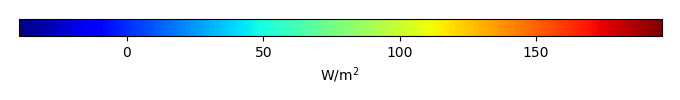MODEL MEANBIAS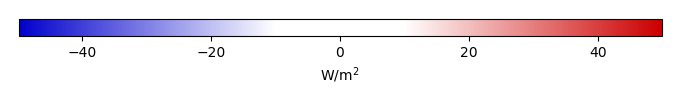BIAS SCORERMSE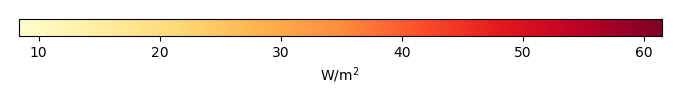RMSE SCOREBENCHMARK INTERANNUAL VARIABILITY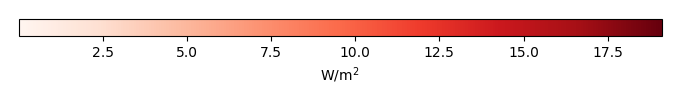MODEL INTERANNUAL VARIABILITYINTERANNUAL VARIABILITY SCOREBENCHMARK MAX MONTHMODEL MAX MONTHDIFFERENCE IN MAX MONTH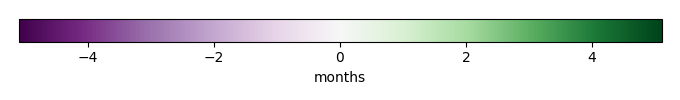SEASONAL CYCLE SCORESPATIAL TAYLOR DIAGRAMMODEL COLORS# Spatially integrated regional mean

MODEL COLORSREGIONAL MEANANNUAL CYCLEMONTHLY ANOMALYANNUAL CYCLE# All Models

BenchmarkCRUNCEPv7GSWP3v1WFDEI# Data Information

creation_date: Thu May 8 22:58:12 PDT 2014

source_file: This product is generated from monthly 1 degree CERES EBAF Radiation observations